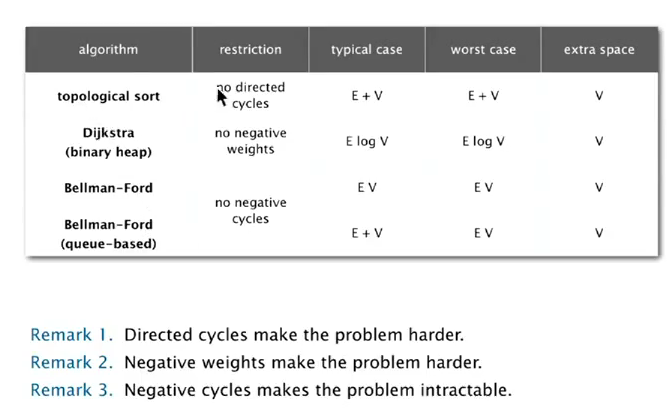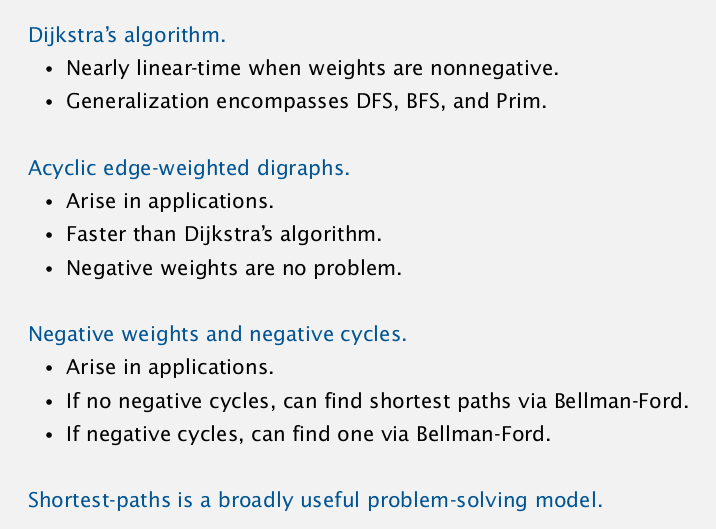# 1. Shortest Paths APIs

context: directe, weighted graphs.

### shortest path variants

in terms of vertices:

• source-sink: form one vertex to another
• single source: from one vertex to all others (considered in this lecture)
• all pairs

constraints on edge weights:

• nonnegative weights
• arbitary weights
• eculidean

cycles:

• no directed cycles
• no negative cycles

### APIs

for weighted directed edge:

```public class DirectedEdge{
DirectedEdge(int v, int w, double weight);
int from();
int to();
double weight();
}
```

for edge-weighted digraph:

```public class EdgeWeightedDigraph{
EdgeWeightedDigraph(int V);
int V();
}
```

for single source shortest-path:

```public class SP{
SP(EdgeWeightedDigraph G, int s);//s is the source node
double distTo(int v);//dist from s to v
Iterable<DirectedEdge> pathTo(int v);// shortest path from s to v
}
```

# 2. Shortest path properties

goal: single-source shortest path
prop. a shortest-path-tree (SPT) exists.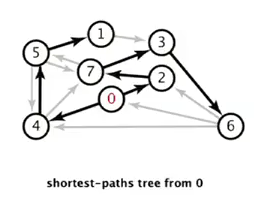⇒ consequence: can represent this SPT by 2 vertex-indexted arrays:

• `double distTo[v]` = shortest path length s → v
• `DirectedEdge edgeTo[v]` = last edge to v in shortest path, `edgeTo[s] = null`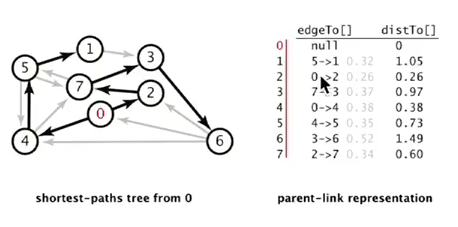```private int distTo[] = new int[V];
private DirectedEdge edgeTo[] = new DirectedEdge[V];
public double distTo(int v){
return this.distTo[v];
}
public Iterable<DirectedEdge> pathTo(int v){
Stack<DirectedEdge> path = new Stack<DirectedEdge>();
for(DirectedEdge e = this.edgeTo[v]; e!=null; e = edgeTo[e.from])
path.push(e);
return path;
}
```

### Edge relaxation

dynamic prog:

• distTo[v] = length of known shortest path from s to v
• distTo[w] = length of known shortest path from s to w
• edgeTo[w] = last edge in the known shortest path form s to w

(consider edges one by one)
def. edge e = v-->w relaxes if e.weight+distTo[v] < distTo[w]. → update distTo[w] and edgeTo[w].```private void relax(DirectedEdge e){
int v = e.from(), w = e.to();
if(distTo[w]>distTo[v]+e.weight()){
distTo[w] = distTo[v]+e.weight();
edgeTo[w] = e;
}
}
```

### optimality conditions

prop. optimality conditions
distTo[] is the solution iff:

• distTo[s] = 0
• distTo[v] is the weight of some path from s to v
• for any edge e = v → w, distTo[w] <= distTo[v]+e.weight()

### Generic algo

just relax all edges...prop. the above generic algo gives the SPT from s.

implementations of the algo:

• Dijkstra (nonnegative edge)
• Topological sort (!) (no directed cycles)
• Bellman-Ford (no negative directed cycles)

# 3. Dijkstra's Algorithm

(non-negative edges)

• consider vertices in increasing distance from s.*
• add vertex to the SPT, relax all edges from that vertex.
• each time: take the closest vertex to s that is not in the SPT (ie. whose dist is not determined yet), add the vertex, and relax all its outgoing edges.

prop. Dijkstra works.
pf.
each edge e = v → w is considered exactly once (when vertex v is added to SPT). distTo[w] <= distTo[v]+e.weight() after relaxing e
ineq holds until algo terminates
* distTo[w] decrease monotonely,
* distTo[v] will not change because each time we choose distTo[v] smallest, and all edges non-negative.
* CQFD by optimality condition.

### implementation

Use an `IndexMinPQ` to store vertices.

```public class DijkstraSP{
private int dist[];
private DirectedEdge edgeTo[];
private IndexMinPQ<Integer,Double> pq;
DijkstraSP(EdgeWeightedDigraph G, int s){
this.dist[] = new int[G.V()];
this.edgeTo[] = new DirectedEdge[G.V()];
this.pq = new IndexMinPQ<Integer,Double>(G.V());
for(int v = 0; v<G.V(); v++)  dist[v] = 999999;
dist[s] = 0;
pq.insert(s, 0.0);
while(!pq.isEmpty()){
int v = pq.delMin();
relax(e);// decrease key or insert vertices to pq
}
}//constructor()

private relax(DirectedEdge e){
int v = e.from(), w = e.to();
if(dist[w]<dist[v]+e.weight()){
dist[w] = dist[v]+e.weight();
edgeTo[w] = e;
if(pq.contains(w)) pq.decreaseKey(w, dist[w]);
else pq.insert(w, dist[w]);
}
}//relax()
}//class
```

Dijkstra is in fact a Prim algorithm ! Both are algos that compute a spanning tree.

• in Prim: each time takes the vertex closest to the tree (and is for undirected graph).
• in Dijkstra: each time takes the vertex closest to source s (and is for directed graph).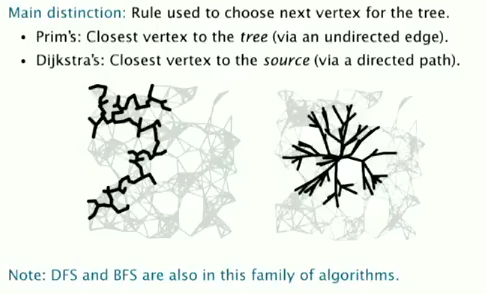DFS BFS are also computing spanning tree!
...NB!!

### complexity

• V insertions to PQ (each vertex is added to the SPT)
• V delMin from PQ
• E decrease key

→ depends on the PQ implementation.
in our implementation, O(ElogV)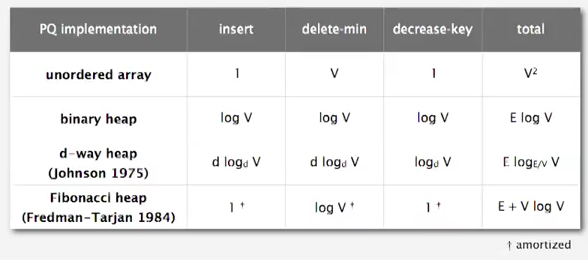# 4. Edge-Weighted DAGs

in a DAG, it's easier to find the shortest path ?
⇒ yes!
simple algo:

• consider vertices in topological order
• relax outgoing edges form this vertex.

### correctness

prop.

topo-sort algo computes SPT in any DAG (even with negative weights) in time O(E+V) (linear time!).

pf.
each edge is relaxed exactely once. distTo[w] <= distTo[v]+e.weight() after relaxing e
ineq holds until the algo terminates because:
* distTo[w] decrease monotonely,
* distTo[v] will not change because of topo-order, no edge pointing to v after v is relaxed.
* CQFD

### implementation

```public class AsyclicSP{
AsyclicSP(EdgeWeightedDigraph G, int s){
// init dist[] and edgeTo[]...
Topological topo = new Topological(G);
for(int v: topo.order())
relax(e);
}
}
```

### application

• seam-carving

Resizing images non-uniformly without distortion.Grid DAG of pixels, edges are pointed to 3 downward neighbors.• longest path in DAG → just negate all edge weights, as this algo is OK for negative edges.

• parallel job scheduling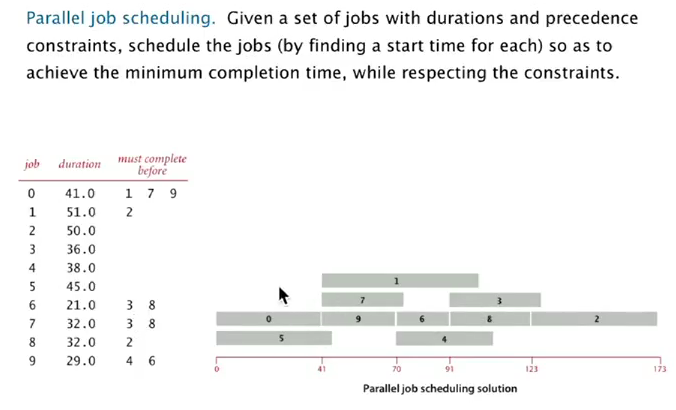感觉有点运筹的意思, 关键是建立一个DAG, 比较有技巧性:

• split each job to 2 vertices (begin and end)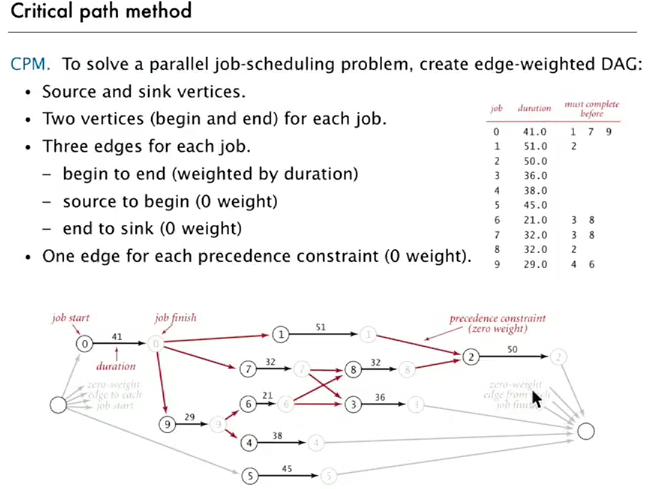⇒ use longest path to schedule jobs

# 5. Negative Weights⇒ need a different algo.

prop. SPT exists iff there is no negative cycles.

### Bellman-Ford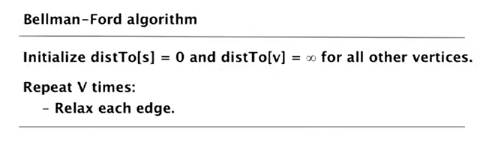(因为relax k次以后, s到v的最短路径的长度小于等于k.)

prop. Bellman-Ford works, complexity is O(EV).
pf.
after i passes, found shortest path contain at most i edges.

code:

```public class BellmanFordSP{
BellmanFordSP(EdgeWeightedDigraph G, int s){
// init dist[] and edgeTo[]...
for(int i=0;i<G.V();i++)
for(DirectedEdge e:G.edges())
relax(e);
}

}
```

### improvement

if dist[v] is not changed during one pasee → no need to relax incoming edges any more.
→ much faster in practice.

FIFO implementation: maintain a queue of vertices whoses dist is changed.

### Finding negative cycle

```boolean hasNegCycle();
Iterable<DirectedEdge> negCycle();
```prop.

if a vertex u is updated in phase V, there exists a negative cycle, and can track edgeTo[u] to find the cycle.

### negative cycle application

arbitrage detection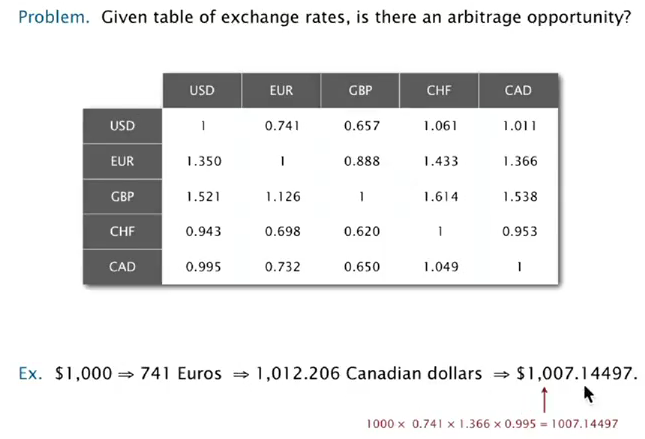find a cycle whose product of edge is >1.
take logs to make a shortest-path pb.
⇒ take minus log, then try to find a negative cycle.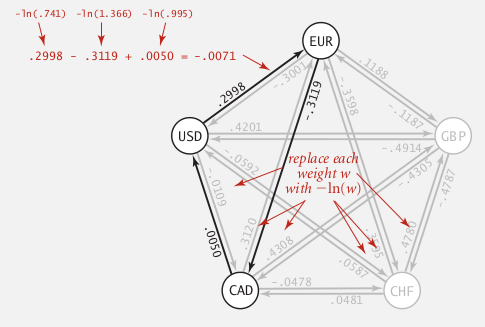NB!!

# Summery